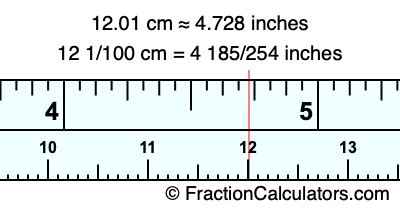12.01 cm in inchesHere is how to convert 12.01 cm to inches. We will give you the fractional answer, the decimal answer, and illustrate the answer on our tape measure.

One centimeter is equal to 50/127 inches, and 12.01 as a fraction is 12 1/100. Therefore, to get the fractional answer to 12.01 cm in inches, we multiply 12 1/100 by 50/127 and simplify if necessary.

cm × 50/127 = inches
12 1/100 × 50/127 = inches
12 1/100 × 50/127 = 4 185/254
12 1/100 cm = 4 185/254 inches
12.01 cm = 4 185/254 inches

As promised above, we also have the answer to 12.01 cm in inches in decimal form. Although the fractional answer above is exact, the decimal answer may be rounded if necessary:

4 185/254 ≈ 4.72834645669291
4 185/254 ≈ 4.728
12.01 cm ≈ 4.728 inches

Our image below shows 12.01 cm on a tape measure. The top row of the tape measure is inches, and the bottom row is centimeters.As you can see, we drew a red line where 12.01 cm and 4.728 inches meet on the measuring tape.

Centimeters to Inches Calculator
Here you can convert another length in centimeters to inches.

Convert  cm to inches.

What is 12.02 cm in inches?
Here is another inches to centimeters calculation we did for you.